### Introduction

This article will cover as to how to make a simple 4 LED serial lighting system using the Arduino Uno programmed from the computer. I would request you to go through our previous articles on the Arduino Uno and assume you have already installed the requisite programming software of Arduino from the official website of Arduino, whose links I have provided below.

### Things Required:

1. Arduino Uno and USB cable – 1 unit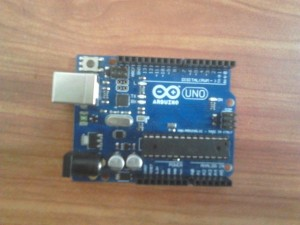2. Light Emitter Diodes (LEDs) – 4 units

3. Resistors (1k ohm) – 4 units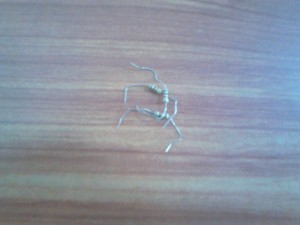4. Wires – As applicable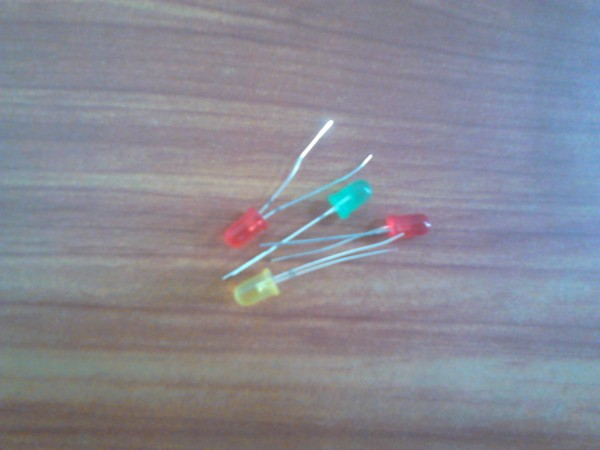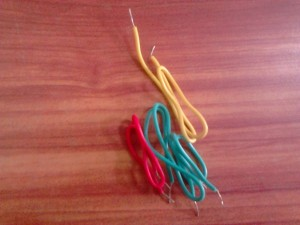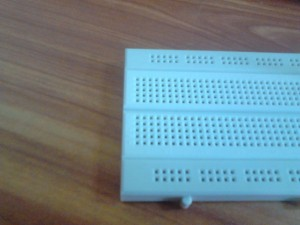### Circuit Diagram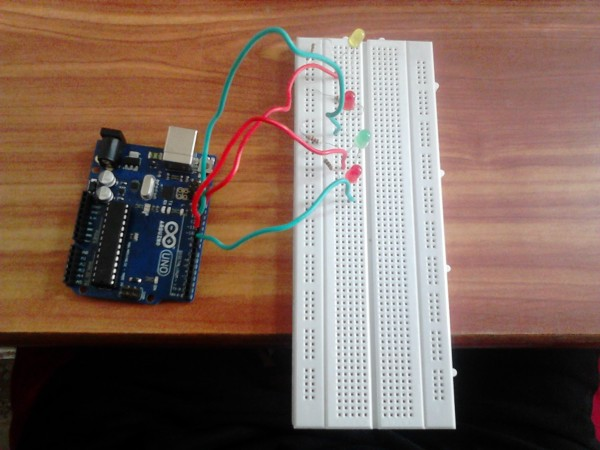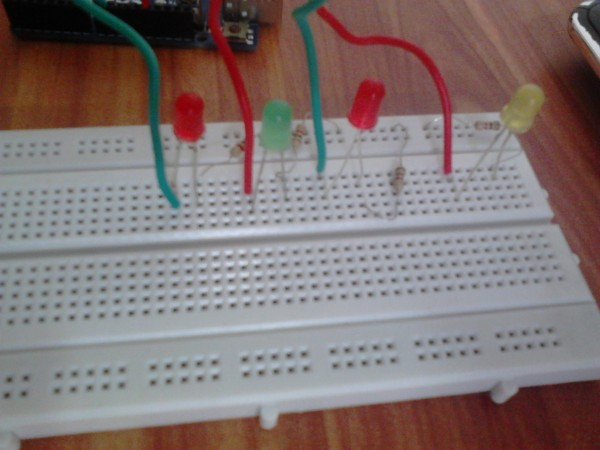1. Connect the LEDs into pins 13, 11, 10 and 9 in series with a resistor each. Note that the longer leg of the LED is the positive terminal and the shorter leg becomes the negative terminal.

2. Complete the circuit by attaching the end of the resistor legs to the GND pin of the Arduino Uno.

### Code

int led_pin = 9;
int led_pin1 = 10;
int i = 0;
int brightness = 5;

void setup()
{
pinMode(led_pin,OUTPUT);
digitalWrite(led_pin,HIGH);
delay(1000);

}

void loop()
{
for(i=0;i<255;i=i+5)
{
analogWrite(led_pin, i);
delay(30);
}
for(i=255;i>=0;i=i-5)
{
analogWrite(led_pin,i);
delay(30);
}
for(i=0;i<255;i=i+5)
{
analogWrite(led_pin1, i);
delay(30);
}
for(i=255;i>=0;i=i-5)
{
analogWrite(led_pin1,i);
delay(30);
}

for(i=0;i<255;i=i+5)
{
analogWrite(11, i);
delay(30);
}
for(i=255;i>=0;i=i-5)
{
analogWrite(11,i);
delay(30);
}
}

### Operation

1. Plug the USB cable into the Arduino Uno and the computer USB port and select the ‘Upload’ Button on the Arduino GUI.

2. You will notice the Tx (Transmitting) and Rx (Receiving) LEDs on the Arduino Uno board blinking, after which you can see the 4 LEDs burning one by one.

You could see my working LED serial display below.

### Possible Extensions and Improvements

1. You could extend this by increasing the number of LEDs on the side, making it an attractive pattern.
2. You could also think of trying to glow/dim the LED in steps.Make modifications in the code for the same.

Share.1.